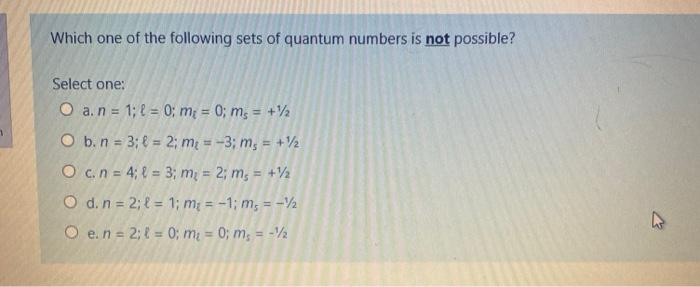Create an Account

Home / Questions / Which one of the following sets of quantum numbers is not possible? Select one: O a. n = 1...

Which one of the following sets of quantum numbers is not possible? Select one: O a. n = 1; f = 0; m = 0; ms = +12 O b. n = 3; 4 = 2; me = -3; ms = +12 O c. n = 4; l = 3; m = 2; ms = +12 O d. n = 2

Which one of the following sets of quantum numbers is not possible? Select one: O a. n = 1; f = 0; m = 0; ms = +12 O b. n = 3; 4 = 2; me = -3; ms = +12 O c. n = 4; l = 3; m = 2; ms = +12 O d. n = 2; l = 1; m:= -1; m, = -2 O e. n = 2:1 = 0; mi = 0; m = -2May 15 2021 View more View LessSubscribe To Get Solution